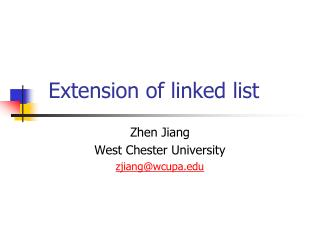# Extension of linked list - PowerPoint PPT PresentationDownload PresentationDownload Presentation- - - - - - - - - - - - - - - - - - - - - - - - - - - E N D - - - - - - - - - - - - - - - - - - - - - - - - - - -
##### Presentation Transcript

1. Extension of linked list Zhen Jiang West Chester University zjiang@wcupa.edu

2. Outline • Double Cyclic Linked List • Queue & Stack • Binary Tree • Expression Tree • Networks

3. (Single) Linked list node NULL

4. Double Cyclic Linked List node NULL

5. Queue node Delete an item from the tail NULL Insert a new item at the head

6. Stack node NULL Insert a new item at the head Delete an item from the head

7. Implementation of stack with template • http://www.cs.wcupa.edu/~zjiang/csc513_template.ppt

8. Use of Stack • Project 5 • Reading information via file • http://www.cs.wcupa.edu/~zjiang/530_proj5_file.pdf • Three stacks, one for each {}, (), and []. • Left => push/insert • Right => pop/delete the closest left symbol • Evaluation of (postfix) expression

9. (5 + 4) * 3 • 5 * (4 + 3) • 5 * 4 + 3 • 5 + (4 + 3) • 5 4 + 3 * • 5 4 3 + * • 5 4 * 3 + • 5 4 3 * +

10. Read the formula from left to right • Number => push • Binary operator => 2 pops • Right number popped first • Then, left number popped. • Calculate result = <second> op <first> • Push (result) • Until expression reading finishes and only one (final) result is left in stack

11. Disorder of the link connection node NULL

12. Tree • Definition • Each node u has n(u) children • Considering the parent-child relationship is undirected, there is no cycle in a tree • There is only one node called the root in the entire tree. It has children only but no parent.

13. Binary Tree • Definition • Definition of tree (3) • For each node u, n(u)<3 • L<S<R • < is a relation between any two nodes in the tree

14. Constructor • Assume each node has a number value so that we have the relation < • Given a sequence: n1, n2, n3, …, ni, … • n1 -> set root unit (node) • ni -> call insertion(node, ni)

15. Insertion(p, n) • If (p->v < n) • If p->R not empty • Insertion (p->R, n) • else • Set p->R • If (p->v > n) • Similar to the case (p->v < n)

16. Travel (print out all node values) • Infix travel, L->S->R • Postfix travel, L->R->S • Prefix travel, S->L->R

17. Infix travel • If node is not empty, Infix_print (node); • Infix_print (p) • If (p->L) infix_print(p->L) • Cout << p->v • If(p->R) infix_print (p->R)

18. Search • If node is not empty, Search (node, n); • Search (p, n) • If (p->v == n) return true • If (p->v < n) return Search (p->R, n) • If (p->v > n) return Search (p->L, n) • Terminating condition: if (!p) return false

19. Deletion • Delete the root node • If node is empty • If node->L (or node->R) is empty • tmp = node->R (or node->L) • Delete node • node = tmp

20. If neither node->L nor node->R is empty • If node->L->R is empty • node -> v = node->L->v • tmp = node->L->L • Delete node->L • node->L = tmp • Else • tmp = node->L • While (tmp->R->R) tmp = tmp->R • node->v = tmp->R->V • tmp2 = tmp->R->L • Delete tmp->R • tmp->R = tmp2

21. See if you can find a certain value in the tree and delete it! • Delete (n) • If (node->v == n) delete node; • If (node->v < n) • deletion (node, node-R, n, 1) • If (node->v > n) • deletion (node, node-L, n, 0)

22. Deletion (parent, start, value, flag) • If start is empty // not found, done! • If start->v < value • If start->v > value • Else //start->v == value • If both start->L and start->R are empty • Delete start • If (flag)Parent ->R = NULL • Else Parent -> L = NULL • If start->L (or start->R) is empty

23. Deletion (parent, start, value, flag) • Else //start->v == value • If start->L (or start->R) is empty • If (flag) Parent-R = Start->R (or start->L) • Else Parent->L = Start->R • Delete start • If neither start->L nor start->R is empty

24. If start->L->R is empty • start -> v = start->L->v • tmp = start->L->L • Delete start->L • start->L = tmp • Else • tmp = start->L • While (tmp->R->R) tmp = tmp->R • start->v = tmp->R->V • tmp2 = tmp->R->L • Delete tmp->R • tmp->R = tmp2

25. Expression Tree • Definition • Definition of binary tree (5) • All leaves are numbers, and all intermediate nodes are operators • To simplify the discussion in this class, we use binary operators only.

26. Evaluation • If node is not empty, R_E(node); • R_E(p) • If p->v is not digit • Lvalue = R_E(p->L); • Rvalue = R_E(p->R); • Return Lvalue <p->v> Rvalue; • Else • Return p->v

27. Print • Postfix • Construction • From infix format • http://www.cs.wcupa.edu/~zjiang/csc530_expressionTree.htm

28. Network • Graph table • http://www.cs.wcupa.edu/~zjiang/csc530_graph_table.pdf • Depth first search • http://www.cs.wcupa.edu/~zjiang/csc530_depth.pdf • Width first search • http://www.cs.wcupa.edu/zjiang/csc530_width.pdf • Shortest path construction • http://www.cs.wcupa.edu/~zjiang/csc530_shortestpath.pdf

29. Spanning (travel) • Project 7